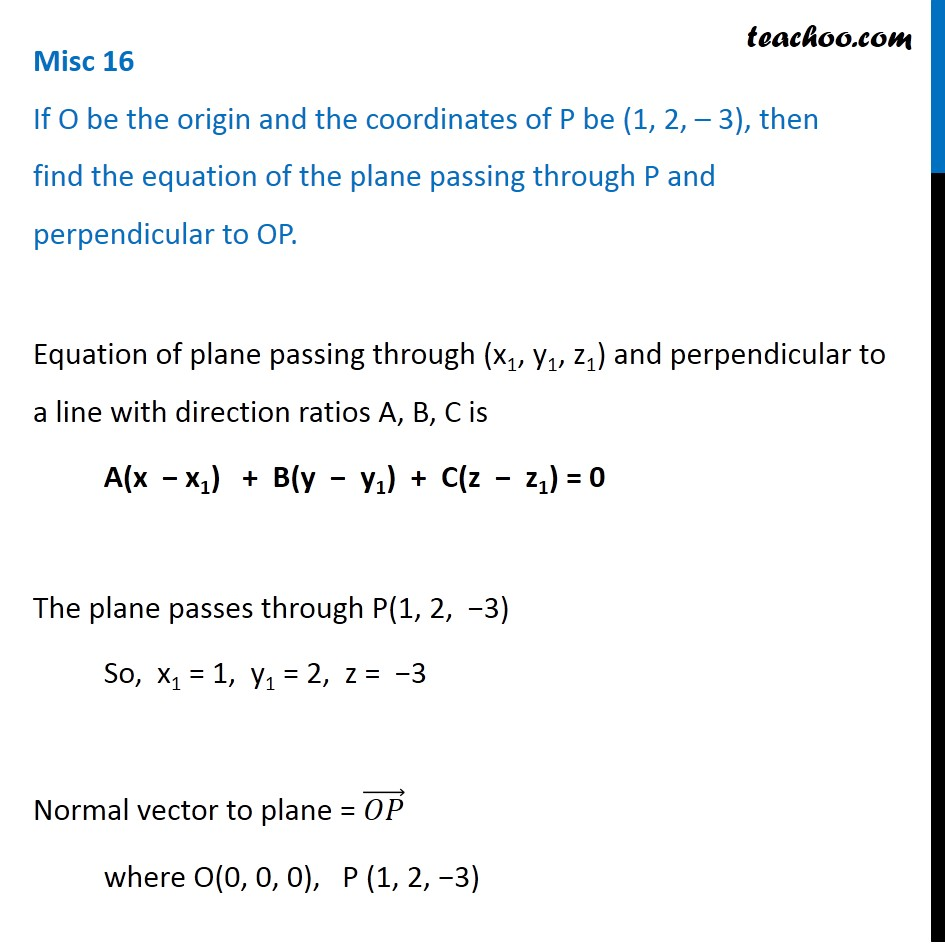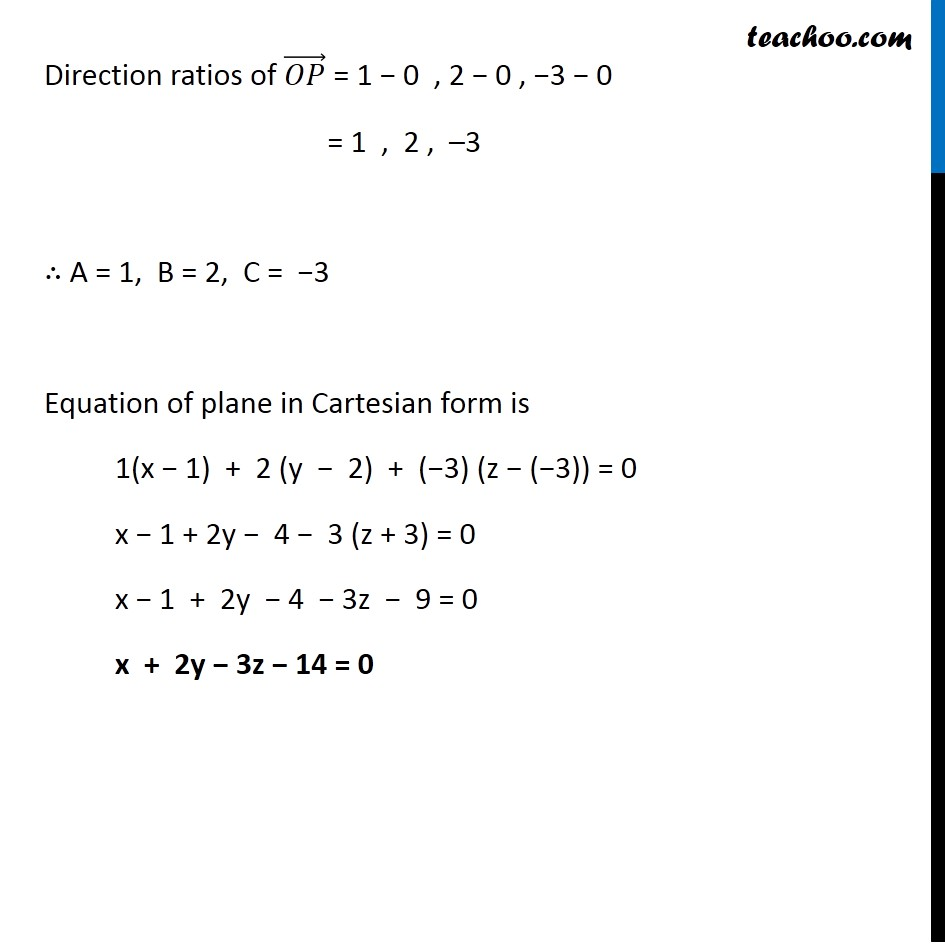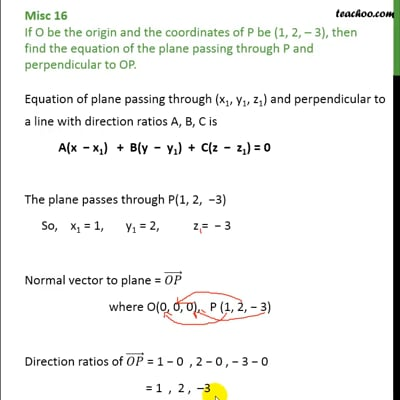Miscellaneous

Chapter 11 Class 12 Three Dimensional Geometry
Serial order wiseThis video is only available for Teachoo black users

Solve all your doubts with Teachoo Black (new monthly pack available now!)

### Transcript

Misc 16 If O be the origin and the coordinates of P be (1, 2, – 3), then find the equation of the plane passing through P and perpendicular to OP.Equation of plane passing through (x1, y1, z1) and perpendicular to a line with direction ratios A, B, C is A(x − x1) + B(y − y1) + C(z − z1) = 0 The plane passes through P(1, 2, −3) So, x1 = 1, y1 = 2, z = −3 Normal vector to plane = (𝑂𝑃) ⃗ where O(0, 0, 0), P (1, 2, −3) Direction ratios of (𝑂𝑃) ⃗ = 1 − 0 , 2 − 0 , −3 − 0 = 1 , 2 , –3 ∴ A = 1, B = 2, C = −3 Equation of plane in Cartesian form is 1(x − 1) + 2 (y − 2) + (−3) (z − (−3)) = 0 x − 1 + 2y − 4 − 3 (z + 3) = 0 x − 1 + 2y − 4 − 3z − 9 = 0 x + 2y − 3z − 14 = 0